# Class-XII Physics:Atomic Structure

Thomson’s Atom Model
According to Thomson, an atom is a sphere of positive charges of uniform density of about 10-10 m diameter in which negative charges i.e. electrons are embedded like plums in the pudding.
Thomson’s model could not explain the presence of discrete spectral lines emitted by hydrogen and other atoms. Hence efforts were made to look for a better model of atom.
Alpha-particle scattering experiment (Geiger—Marsden experiment)
Rutherford along with Geiger and Marsden performed a series of experiments on the scattering of alpha-particles due to atoms of thin gold foils. An alpha-particle is a helium atom from which two electrons have been removed. Thus, its charge is +2e and mass is nearly four times the mass of a proton.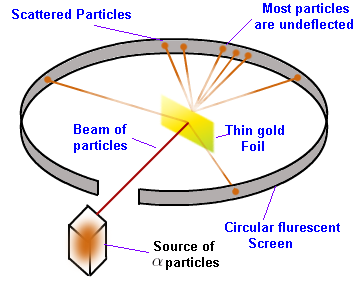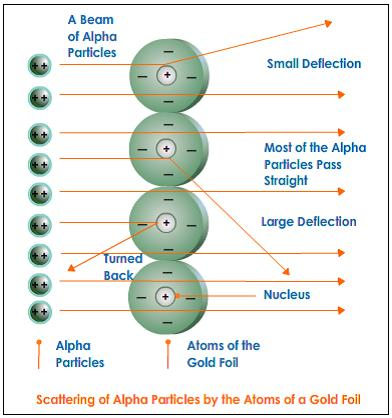Rutherford observed that
(1) Most of the α-particles passed through the gold foil undeflected.
(2) Some of the α-particles were deflected through small angles.
(3) A few α-particles (1 in 9000) were deflected through large angles (may be greater than 90°). Some of them even retraced their path i.e. angle of deflection was 180°.
(4) The number of α-particles per unit area that reach the screen at a scattering angle θ were found to vary as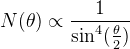The graph between the number of α-particles scattering at an angle θ and the angle of scattering (θ) is shown in Figure .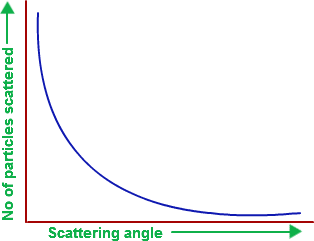Rutherford Nuclear Model of Atom
1. Most of the mass and all the positive charges of an atom are concentrated in a very small region known as atomic nucleus.
2. The size of the nucleus is extremely small  as compared to the size of the atom. So most of the space in an atom is empty.
3. The negatively charged particles known as electrons revolve around the nucleus in the circular orbits.. The centripetal force required for rotating the electrons around the nucleus is provided by the coulomb’s force of attraction between the nucleus and the electrons.
4. The number of revolving electrons is equal to the number of positive charges in the nucleus, hence atom is electrically neutral.
Distance of Closest Approach
The minimum distance upto which an energetic α-particle travelling directly towards the nucleus can move before coming to rest and then retracing its path is known as distance of closest approach.This distance estimates the size of the nucleus.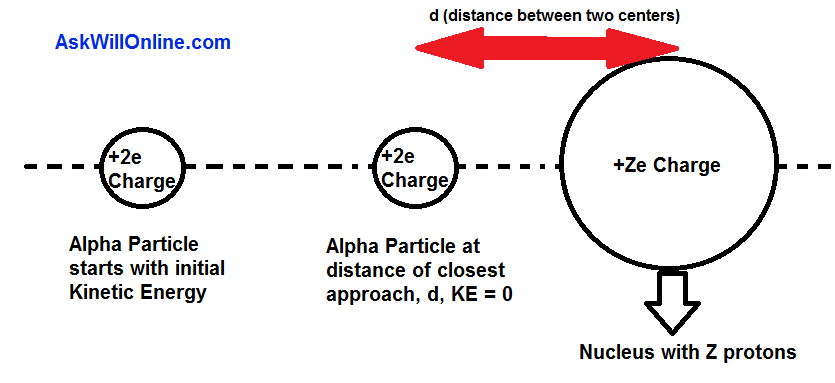The distance of closet approach (d) is given by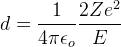where E is the initial energy of α-particle
Z is atomic number
e is the charge of electron
Impact Parameter (b)
The perpendicular distance of the velocity vector (u) of the α-particle from the centre of the nucleus when it is far away from the nucleus is known as impact parameter.It is denoted by b.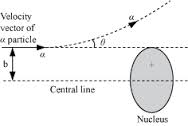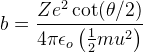Drawbacks of Rutherford’s Model of Atom
(i) It failed to explain the stability of the atom: According to Rutherford, electrons revolve around the nucleus in circular orbits. Anything which moves in a circular path is accelerated towards the centre of the circular path and has centripetal acceleration. Thus, the electrons revolving around the nucleus are accelerated towards the nucleus of the atom. According to classical electrodynamics, any charged particle while accelerating or retarding loses energy through electromagnetic radiations. Therefore, the electron revolving around the nucleus must lose energy continuously. As a result of this, the radius of the path of the electron should go on decreasing and ultimately it is to fall into the nucleus by following a spiral path (as shown in Figure). But, in fact it never happens.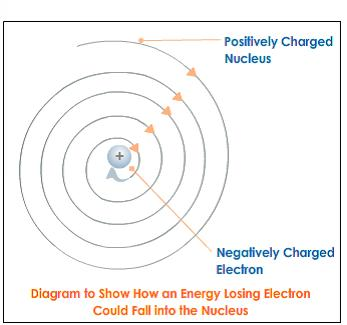(ii) It failed to explain the spectrum of an atom.
Energy Quantization
The energy of a system of particles is quantized (i.e.,have a set of discrete values) if the particles of the system are confined to a certain region of space.
Bohr’s Atom Model
1.  An atom has a small positively charged core where whole of the mass of an atom is supposed to be concentrated. This core is called nucleus of the atom.
2. The electrons revolve round the nucleus in fixed orbits of definite radii. As long as the electron is in a certain orbit, it does not radiate any energy.Therefore, the orbits in which electrons move are called stationary states. Each stationary state or the orbit has a definite amount of energy.
3. The electrons can revolve only in those orbits, in which its angular momentum is an integral multiple of (h/2π).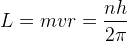i.e. angular momentum of an electron in an atom is quantized.(Bohr’s quantization condition for angular momentum)
where,
L is the angular momentum of electron
m = mass of an electron
v = velocity of an electron,
r = radius of the orbit
h = Planck’s constant
n = 1, 2, 3, is an integer called principal quantum number.
4. The energy is radiated in the form of a photon, only when an electron jumps from higher energy orbit to lower energy orbit.
If Ei and Ef are the energies associated with the orbits of principal quantum numbers ni and nf of respectively (nf > ni), then the amount of energy radiated in the form of a photon is given by
hv = Ei – Ef
• Stationary state does not mean that the electron is stationary but it means that the energy of the electron remains constant as long as it keeps on moving in the same orbi

t.

• When external energy is supplied to the atom, an electron in any orbit absorbs this energy and goes to the higher energy orbit. After 10-8 s, the electron jumps back to the original orbit by emitting the absorbed energy in the form of a photon.
Bohr’s theory of Hydrogen Atom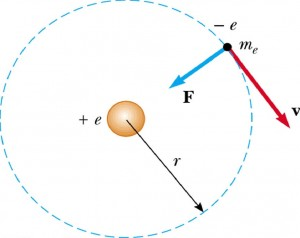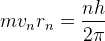———– ( 1 )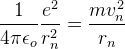———- ( 2 )
Radius of nth orbit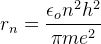———– (3)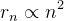radius of 1st orbit (ao)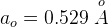Speed of electron in nth orbit——– (4)Speed of electron in 1st orbit (v1)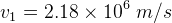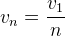Energy of electron
• Kinetic energy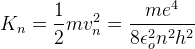——– (5)
• Potential energy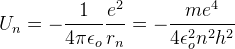——–(6)
• Total energy
En = Kn + Un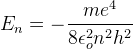——- (7)
Total energy in 1st orbit
E = – 13.6 eV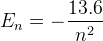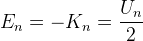### Hydrogen like atoms

They have 1 electron in atom like singly ionised helium He+,doubly ionised lithium Li++.
Therefore,Rydberg formula for hydrogen like atom spectrum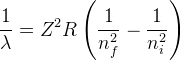where Z = atomic number
Replace,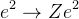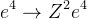in equation 3,4,5,6,7 given above to obtain radius,speed and energies of electron in hydrogen like atoms.Here Z is the atomic number of hydrogen like atoms.
• Radius of nth orbit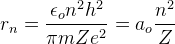• Speed of electron in nth orbit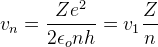Energy of electron
• Kinetic energy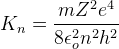• Potential energy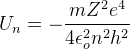• Total energy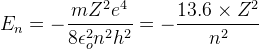Hydrogen Spectrum
Under normal condition electron stays in ground state.After absorbing some energy from external it goes to higher energy state level and stays for about 10-8 seconds and then come back to lower energy state and emits electromagnetic radiation of frequency. This radiation is called spectral line.
The wavelength of the spectral line depends upon the energies between two energy states in which the transition of electron takes place. The spectral lines arising from the transition of electron from the higher energy states to a particular lower energy state form a spectral series as shown in Figure.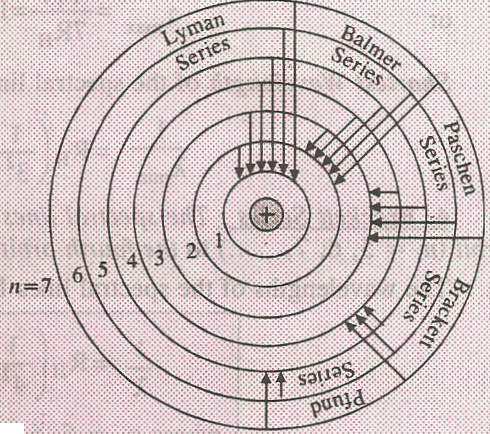Energy level diagram of hydrogen Atom:
The energy of an electron corresponding to each orbit (energy state) can be represented by the horizontal lines as shown in figure below. This is called an energy level diagram. The transitions of electron between the orbits is shown by vertical arrows between the energy levels. The energy of each emitted photon is proportional to the length of the arrow between two corresponding energy levels.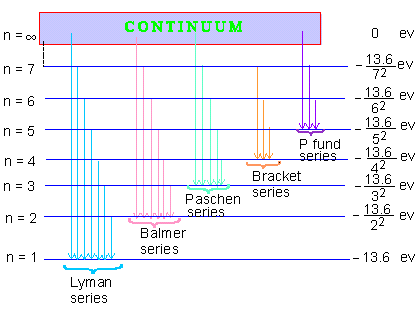## Hydrogen Spectrum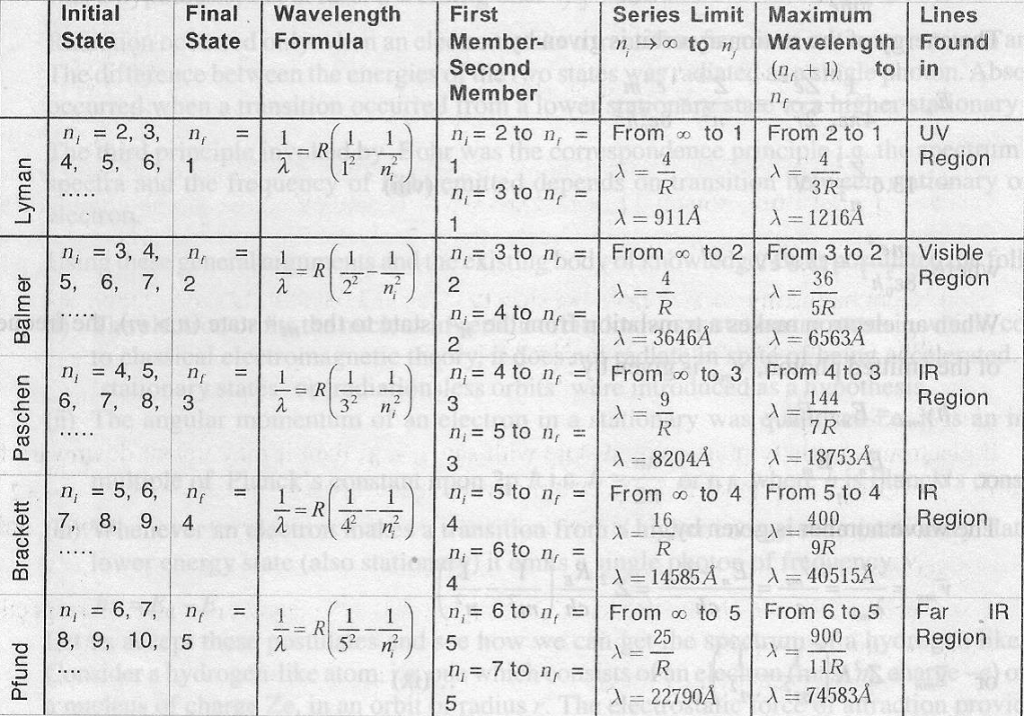Rydberg formula for hydrogen atom spectrum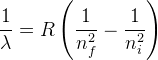where R = Rydberg’s constant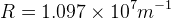(1/λ) is called wave number
1. Lyman Series : The spectral lines emitted due to the transition of an electron from any outer orbit (ni = 2, 3, 4, 5,…. ) to the first orbit (nf = 1) form a spectral series known as Lyman series.
• The wavelengths of the spectral lines forming a Lyman series are given by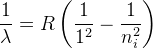• This series lies in the ultra-violet region of the electromagnetic spectrum.
• Longest Wavelength of the spectral line of Lyman series is emitted when the transition of electron takes place from ni = 2 to nf = 1.
• Shortest Wavelength of the spectral line (series limit) of Lyman series is emitted when the transition of electron takes place from ni = ∞ to nf = 1.
2. Balmer Series : The spectral lines emitted due to the transition of an electron from any outer orbit (ni = 3, 4, 5, 6, ….) to the second orbit (nf = 2) form a spectral series known as Balmer series.
• The wavelengths of the spectral lines forming Balmer series are given by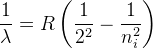• This series lies in the visible region of the electromagnetic spectrum.
• Longest Wavelength of the spectral line of Balmer series is emitted when the transition of electron takes place from ni = 3 to nf = 2.
• Shortest Wavelength of the spectral line (series limit) of Balmer series is emitted when the transition of electron takes place from ni = ∞ to nf = 2.
3. Paschen Series : The spectral lines emitted due to the transition of an electron from any outer orbit (ni = 4, 5, 6,…. ) to the third orbit (nf = 3) form a spectral series known as Paschen series.
• The wavelengths of the spectral lines forming Paschen series are given by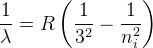• This series lies in the Infra–red region of the electromagnetic spectrum.
• Longest Wavelength of the spectral line of Paschen series is emitted when the transition of electron takes place from ni = 4 to nf = 3.
• Shortest Wavelength of the spectral line (series limit) of Pasc

hen series is emitted when the transition of electron takes place from ni = ∞ to nf = 3.

4. Brackett Series : The spectral lines emitted due to the transition of an electron from any outer orbit (ni = 5, 6, 7, ….) to the fourth orbit (nf = 4) form a spectral series known as Brackett series.
• The wavelengths of the spectral lines forming Brackett series are given by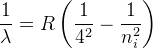• This series lies in the Infra–red region of the electromagnetic spectrum.
• Longest Wavelength of the spectral line of Brackett series is emitted when the transition of electron takes place from ni = 5 to nf = 4.
• Shortest Wavelength of the spectral line (series limit) of Brackett series is emitted when the transition of electron takes place from ni = ∞ to nf = 4.
5. Pfund Series : The spectral lines emitted due to the transition of an electron from any outer orbit (n1 = 6, 7, 8, ….) to the fifth orbit (nf = 5) form a spectral series known as Pfund series.
• The wavelengths of the spectral lines forming Pfund series are given by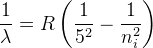• This series lies in the far infra–red region of the electromagnetic spectrum.
• Longest Wavelength of the spectral line of Pfund series is emitted when the transition of electron takes place from ni = 5 to nf = 4.
• Shortest Wavelength of the spectral line (series limit) of Pfund series is emitted when the transition of electron takes place from ni = ∞ to nf = 5.
Limitations of Bohr’s atomic model:
1. It successfully explains the spectra of simple atoms (i.e. the atoms having only one electron). For example, it can explain the spectra of hydrogen atom and hydrogen like atoms (He+, Li+ + etc). But this model could not explain the spectra of complex atoms having more than one electron.
2. When the spectra

l lines of a Balmer series was observed under a powerful microscope, it was found that each spectral line consists of closely spaced lines. Bohr’s model of atom could not explain this fine structure of the spectral line of Balmer series.

3. Bohr’s atomic model does not give any indication regarding the arrangement and distribution of electrons in an atom.
4. This model could not account for the wave nature of electrons.
5. It does not give any idea about the relative intensities of spectral lines.
6. This model could not explain the splitting of spectral line into a number of spectral lines under the effect of magnetic field (Zeeman effect).

This site uses Akismet to reduce spam. Learn how your comment data is processed.

error: Content is protected !!